## Saturday, November 12, 2005

### The Key Theorem of the Learning Theory

The Key Theorem of the Learning Theory is what Vapnik calls his theorem (for instance here) that gives the necessary conditions for the Empirical Risk Minimization principle to be consistent.
The important equation is the following: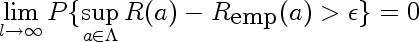Here I'll explain the parts of the formula using the following motivating example:
Suppose our true distribution is a normal distribution with unit variance, centered at 0, and we estimate its mean from data using method of maximum likelihood. This falls into domain of Empirical Risk Minimization because it's equivalent to finding unit variance normal distribution which minimizes empirical log-loss. If this method is consistent, then our maximum likelihood estimate of the mean will be correct in the limit

In order for the theorem to apply, we need to do make sure that we can put a bound on the loss incurred by functions in our hypothesis space, so here, make an additional restriction that our fitted normals must have mean between -100 and 100.

Here's what parts of the equation stand for

1.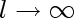l is the size of our training set

2.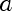a is the parameter that indexes our probability distributions, in this case, it's mean of our fitted Normal. Lambda is it's domain, in this case,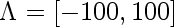3.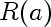This is the actual risk incurred by using estimate a instead of true estimate (0). In our case the loss is log loss, so the risk is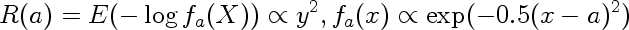In other words it is the expected value of the negative logarithm of the probability assigned to observations when observations come from Normal centered at 0, but we think they come from Normal centered on a

4.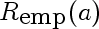This is the risk incurred by using estimate a, estimated on a finite sample drawn from the true density. In our case it is the following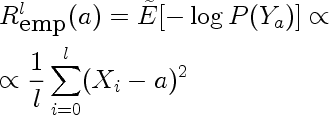Because the sample is a random variable, empirical risk is also a random variable. We can use the method of transformations to find it's distribution. Here are distributions for empirical risk estimated from training sets of size one for different values of a.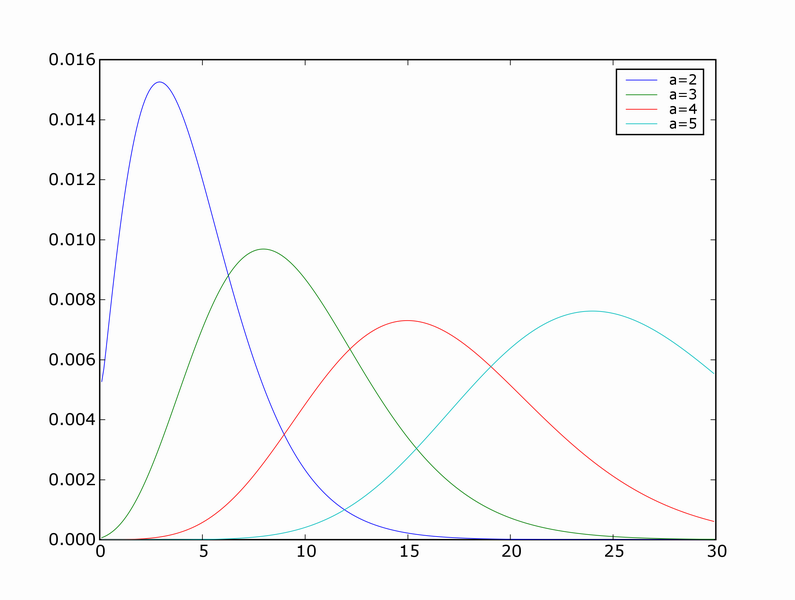You can see that the modes of the distributions correspond to the true risk (a^2), but the means don't.

5.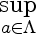Supremum is defined as the least upper bound, and behaves lot like maximum. It is more general than the maximum however. Consider the sequence 0.9, 0.99, 0.999, ....
This sequence does not have a maximum but has a supremum.

6.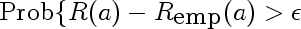This is the simplified middle part of the equation. It represents the probability of our empirical risk underestimating true risk by more than epsilon, for a given value of a. This would be used in place of the supremum if we cared about pointwise as opposed to uniform, convergence. Intuitively, we expect this probability to get smaller for any fixed epsilon as the sample size l gets larger. Here are a few densities of risk distribution for a=4 and different values of l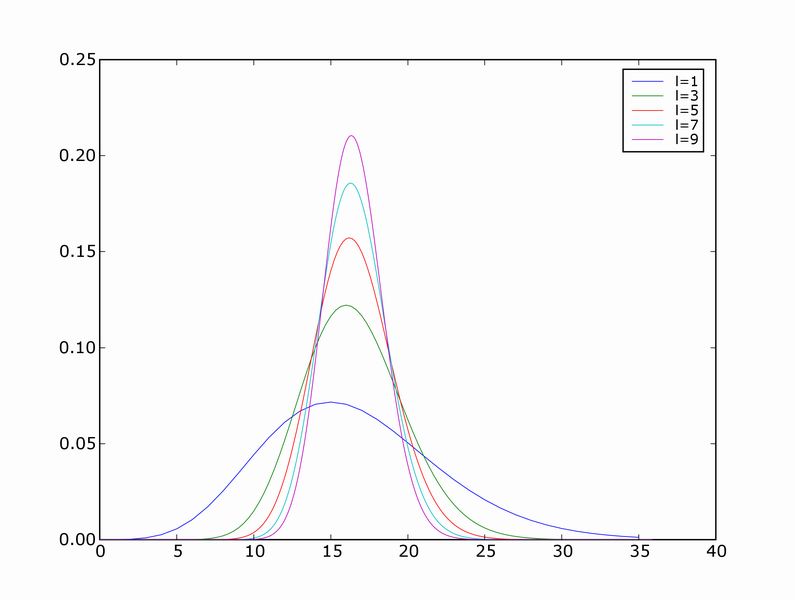7.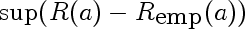This is the random variable that represents the largest deviation of the empirical risk from corresponding true risk, over all models a. It's like an order statistic, except the samples are not IID, and there are infinitely many of them.

Questions:
• How can one find the distribution of \sup(R(a)-R_emp(a) in the setting above?
I came across an interesting article by Zhu and Lu talking about how non-informative priors can often be counter-intuitive. For instance suppose we are estimating parameter of a Bernoulli distribution. If we don't know anything, the most intuitive prior to use is uniform. However Jeffreys' "uninformative" prior doesn't look uniform at all:

#### 8 comments:

Connelly said...

Hi Yaroslav.

I'm learning Bayesian nets in CS 430 -- it's fun. I just wanted to say "hi" on your blog. Hope you're having fun in school.

P.S.: If you haven't seen it yet, the Banach fixed point theorem gives a nice test for convergence of iterated functions.

Joseph Ferguson said...

Some of people do the post aicm now and they do it before usmle. It is really surprising that they choose this first and it could be tough at beginning but they can easily get it later. nice article & it will give you some amazing idea and very effective for the papers writing.

bollings said...

While I was going to learn some theory it really gives me pain as ther ehas hardship but here are some tips which help me out. website you'll be find some helpful information about the academic writing service.

nill said...

There has nothing special you can do in the theory side if you do not study and try to practice it in your own way at home. look here for the students that is very helpful for the writing services.

explore the details said...

My best friend send me the link and told me that you go to the page and visit there. Because this page share their post for the people like you. That's why I'm here. SO good really. Thanks to my friend for this link.

naina said...

I am weak in The Key Theorem of the Learning Theory but I am very well with graf. I see you posted both of information here. its impresed me. I will check more info from your website in future. thanks

draj said...

Excellent machine learning blog,thanks for sharing...
Seo Internship in Bangalore
Smo Internship in Bangalore
Digital Marketing Internship Program in Bangalore

draj said...

Excellent machine learning blog,thanks for sharing...
Seo Internship in Bangalore
Smo Internship in Bangalore
Digital Marketing Internship Program in Bangalore